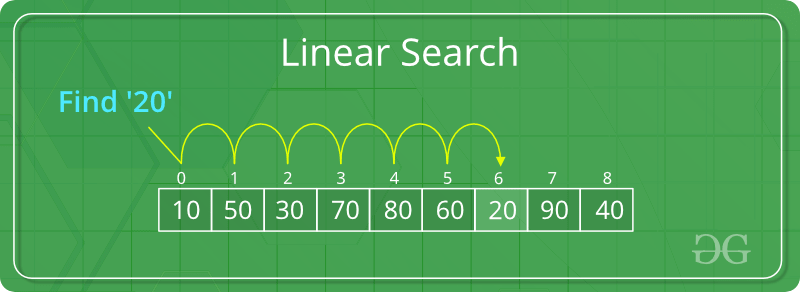# Python | Linear search on list or tuples

Let’s see a basic linear search operation on Python list and tuples.

A simple approach is to do linear search, i.e

• Start from the leftmost element of list and one by one compare x with each element of the list.
• If x matches with an element, return True.
• If x doesn’t match with any of elements, return False.Example #1: Linear Search on Lists

 `# Search function with parameter list name ` `# and the value to be searched ` `def` `search(``list``,n): ` ` `  `    ``for` `i ``in` `range``(``len``(``list``)): ` `        ``if` `list``[i] ``=``=` `n: ` `            ``return` `True` `    ``return` `False` ` `  `# list which contains both string and numbers. ` `list` `=` `[``1``, ``2``, ``'sachin'``, ``4``,``'Geeks'``, ``6``] ` ` `  `# Driver Code ` `n ``=` `'Geeks'` ` `  `if` `search(``list``, n): ` `    ``print``(``"Found"``) ` `else``: ` `    ``print``(``"Not Found"``) `

Output:

```Found
```

Note that list are mutable but tuples are not.

Example #2: Linear Search in Tuple

 `# Search function with parameter list name ` `# and the value to be searched ` `def` `search(``Tuple``, n): ` ` `  `    ``for` `i ``in` `range``(``len``(``Tuple``)): ` `        ``if` `Tuple``[i] ``=``=` `n: ` `            ``return` `True` `    ``return` `False` ` `  `# list which contains both string and numbers. ` `Tuple``=` `(``1``, ``2``, ``'sachin'``, ``4``, ``'Geeks'``, ``6``) ` ` `  ` `  `# Driver Code ` `n ``=` `'Geeks'` ` `  `if` `search(``Tuple``, n): ` `    ``print``(``"Found"``) ` `else``: ` `    ``print``(``"Not Found"``) `

Output:

```Found
```

My Personal Notes arrow_drop_upCheck out this Author's contributed articles.

If you like GeeksforGeeks and would like to contribute, you can also write an article using contribute.geeksforgeeks.org or mail your article to contribute@geeksforgeeks.org. See your article appearing on the GeeksforGeeks main page and help other Geeks.

Please Improve this article if you find anything incorrect by clicking on the "Improve Article" button below.